Your browser doesn't support the features required by impress.js, so you are presented with a simplified version of this presentation.

For the best experience please use the latest Chrome, Safari or Firefox browser.

CIS 115

Cryptography

Message to the Class

``TSTEPHAAXLISLAESCEMQIYQ``

ScytaleImage Source: Wikipedia

Message to the Class

``TSTEPHAAXLISLAESCEMQIYQ``
``T    H    I    S    I``
`` S    A    S    C    Y``
``  T    A    L    E    Q``
``   E    X    A    M``
``    P    L    E    Q``

Early Ciphers

• Substitution Ciphers
• Cryptoquip - Easily Breakable
• Polyalphabetic Ciphers
• First described by Al-Kindi in the 9th century
• Later explained by Leon Battista Alberti in 1467

Tabula Recta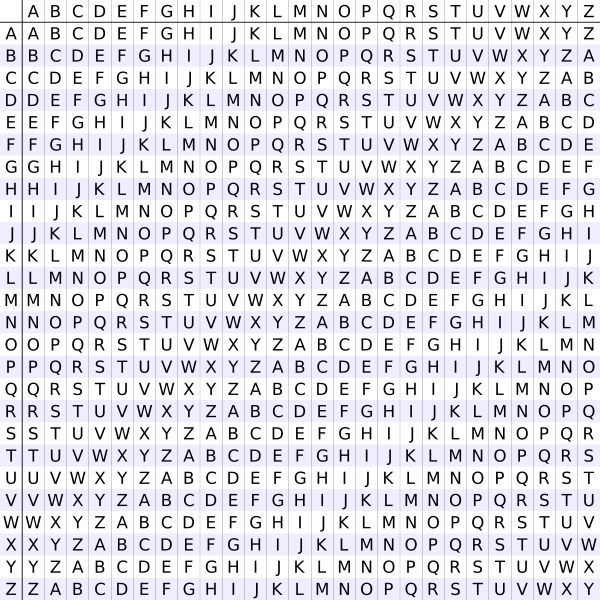Image Source: Wikipedia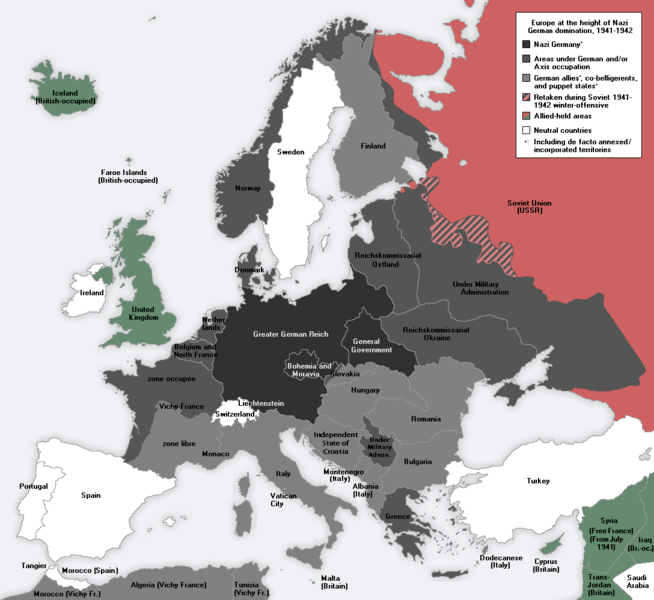Image Source: Wikipedia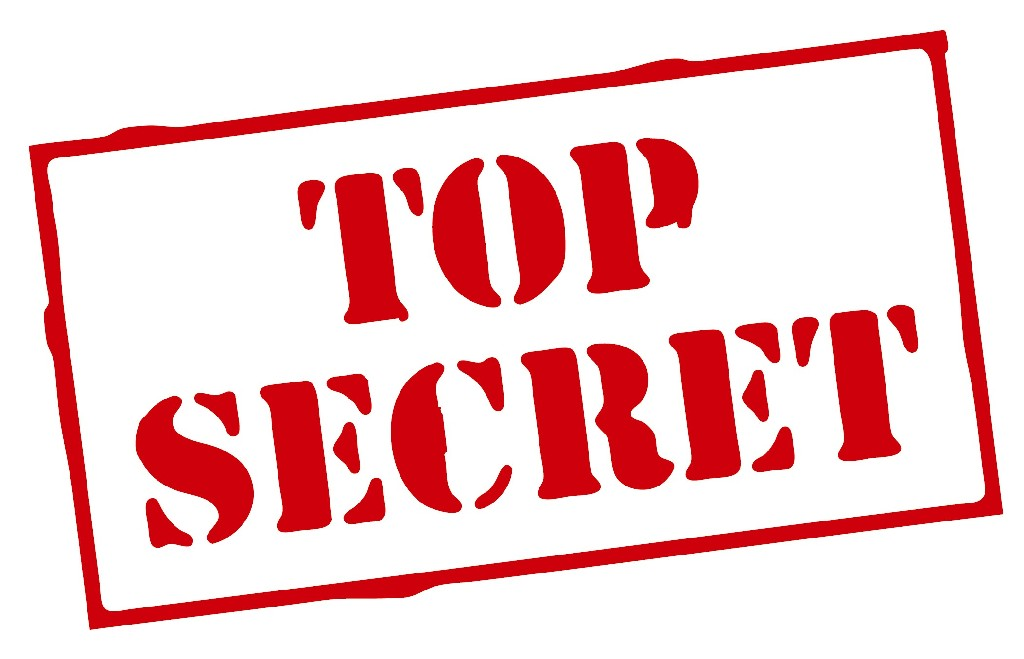Enigma MachineImage Source: Wikipedia

Enigma Machine Rotors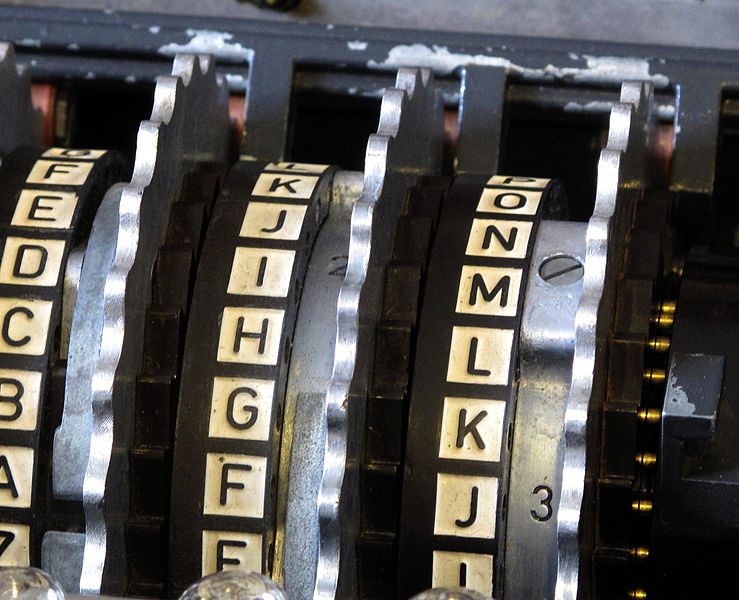Image Source: Wikipedia

Enigma Machine Rotors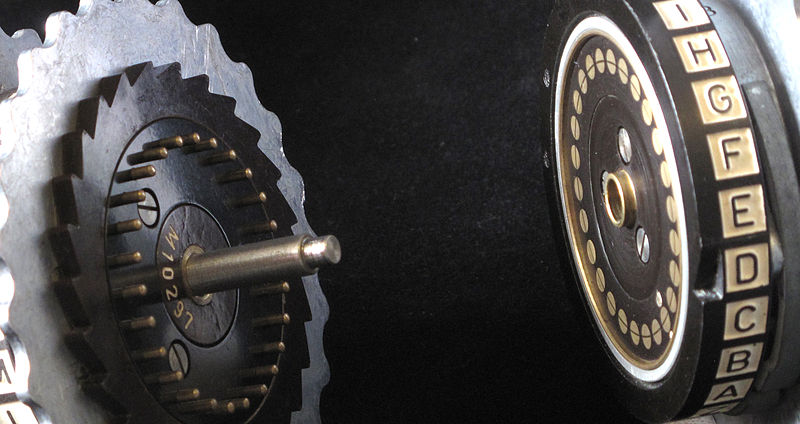Image Source: Wikipedia

Enigma Machine Ratchet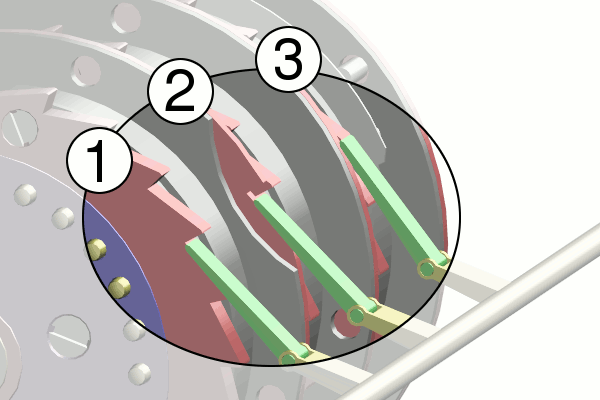Image Source: Wikipedia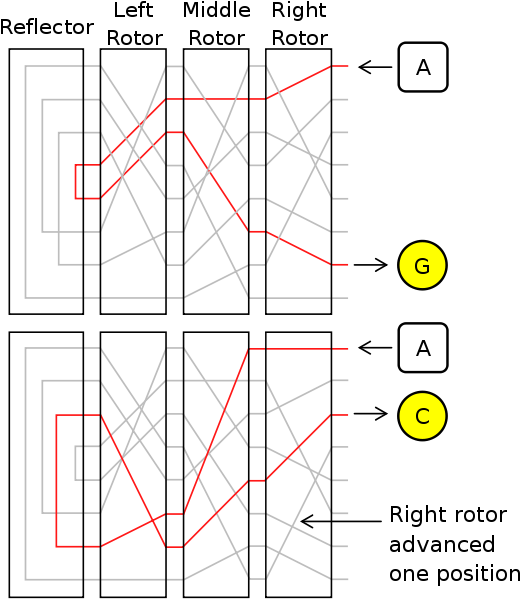Image Source: Wikipedia

Enigma Machine Plugboard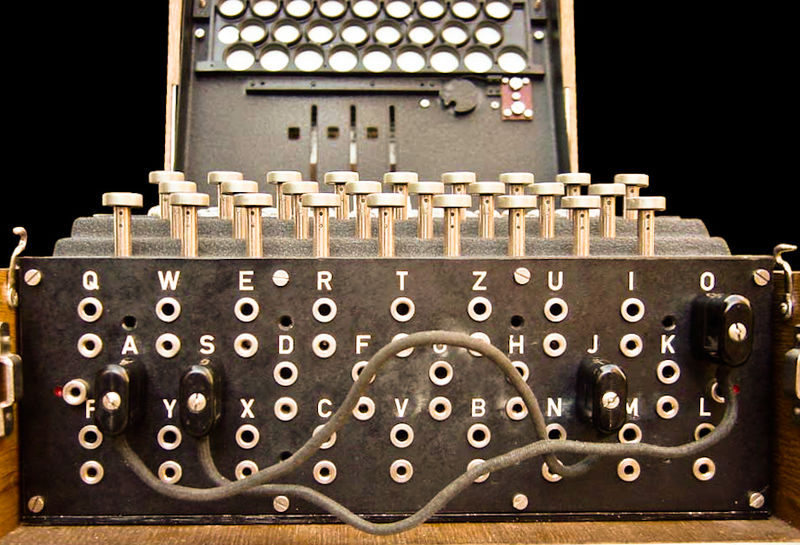Image Source: Wikipedia

Enigma Key

• Choice and order of rotors
• Initial position of rotors
• Ring setting on rotors
• Plug connections

Enigma Operation

• Set wheels to today's key from codebook
• Operator chooses message key
• Encode message key TWICE to avoid errors
• Set wheels to message key
• Encrypt and send message

Enigma Stengths

• Many factors to the encryption
• Had up to 8 different wheels to choose from by the end of the war
• 150 Trillion different setups

Enigma Weaknesses

• A letter would never encrypt to itself
• Plugboards were reciprocal
• Wheels were not similar enough (could determine which wheels were used)
• Poor policies and procedures

Marian Rejewski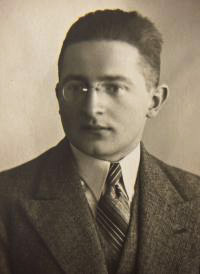Image Source: Wikipedia

Cracking Enigma

• 1932 - First cracked by Marian Rejewski of Poland
• 1938 - Germany added 2 wheels
• 1939 - Alan Turing creates Bombe
• 1945 - Almost every message deciphered within 2 days

Bombe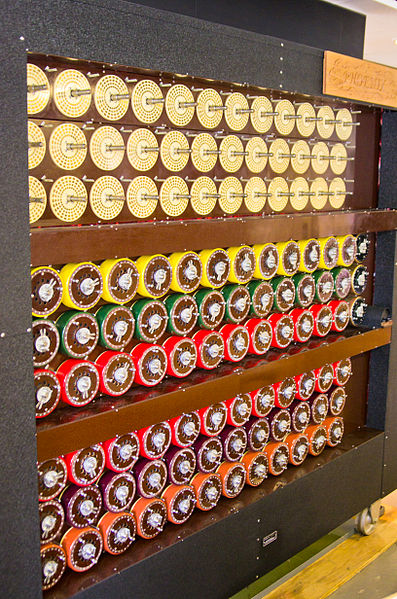Image Source: Wikipedia

Impact

“My own conclusion is that it shortened the war by not less than two years and probably by four years … we wouldn't in fact have been able to do the Normandy Landings, even if we had left the Mediterranean aside, until at the earliest 1946, probably a bit later.”

-Sir Harry Hinsley
British Intelligence Historian

Claude Shannon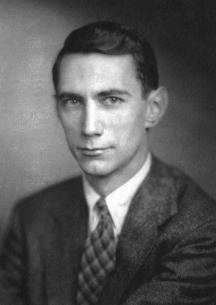Image Source: Wikipedia

Symmetric Key Encryption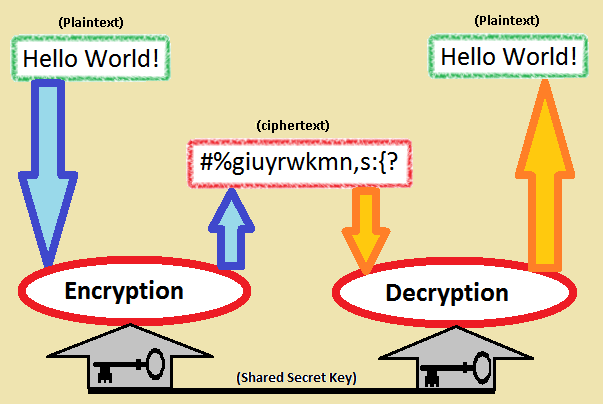Image Source: Wikipedia

Public Key Encryption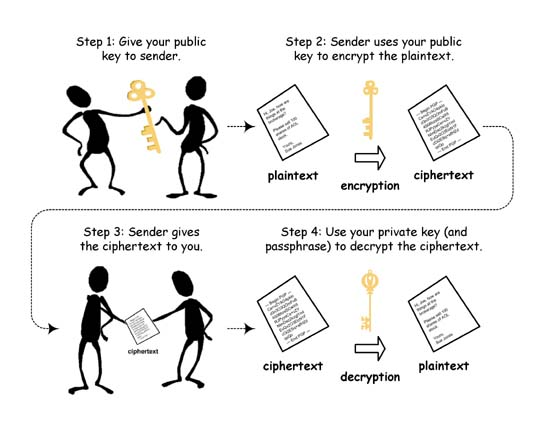RSA Encryption

• Developed in 1977
• Named for the 3 creators (Ron Rivest, Adi Shamir, Lenonard Adleman)
• Uses the product of 2 large prime numbers to generate a key
• Key strength depends on the difficulty of factoring large numbers

RSA Example

• Choose 2 distinct prime numbers
p and q
• Compute their product n = pq
• Compute the totient t of n:
t = (p - 1)(q - 1)

RSA Example

• Choose any number e less than t that is coprime to t (they share no common factors but 1)
• Calculate d as the modular multiplicative inverse of e (mod t)
e * x = 1 (mod t)

RSA Keys

• Public Key : (n, e)
• Encode: c = me (mod n)

• Private Key : (n, d)
• Decode: m = cd (mod n)

Assignments

• Read and be prepared to discuss:
• 9ALG 5: Error Correcting Codes
• Blog 6 - Due 4/18 10:00 PM
• Wiki Draft - Due 4/15 10:00 PM

Blog 6: Computing Science & Mathematics

As we embark on reading the last textbook, it should be very obvious by now that mathematics is a core aspect of Computing Science. In fact, most Computing Science departments and disciplines have a direct foundation in the study of mathematics. Here’s a good quote summing it up from Harold Abelson: “Mathematics provides a framework for dealing precisely with notions of "what is". Computation provides a framework for dealing precisely with notions of "how to".” For this article, write about how Computing Science and Mathematics are related. (Food for thought - Read up on Theoretical Computer Science and Applied Mathematics: https://en.wikipedia.org/wiki/Theoretical_computer_science and https://en.wikipedia.org/wiki/Applied_mathematics). Things you can talk about:

• What mathematical concepts are you most interested in? How do they relate to Computing Science?
• Which algorithms do you think are most closely tied to mathematics? Which ones only make sense when applied to a computer?
• What areas of theoretical computer science do you find most interesting? Why are those interesting to you?
• What have you read in the textbooks so far that relates closely to mathematics?
• Who are some major historical figures that are important to both mathematics and Computing Science? Why do you think they are important in both fields?